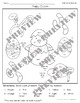# March - April Color by Number - Addition and SubtractionSubject
Resource Type
File Type

PDF

(9 MB|16 pages)
Standards
Also included in:
1. This Color by Addition and Subtraction Year Long bundle will contain 60 total color by number sheets. One third of the sheets will focus on addition to 10,one third on addition to 20 and one third on Subtraction from 20. This bundle includes all my Color by Number Addition and Subtraction Sets. Buyi
\$18.75
\$15.00
Save \$3.75
• Product Description
• StandardsNEW
March - April Color By Number Addition and Subtraction Set. This set contains 12 Color By Number pages including Spring, St. Patrick's Day, April Fools Day, Basketball Champions, Reading Day, and Easter pages.

4 pages focus on Addition to 10, 4 pages focus on Addition to 20, and 4 pages focus on Subtraction from 12.

What's Included:

Spring and March/April Holiday Pages Included
Rainy Days – Addition to 10
Signs of Spring – Addition to 20
Spring Flowers – Subtraction
Irish Symbols – Addition to 20
St. Patrick’s Day Friends – Subtraction
April Fools Day – Addition to 10
All Kinds of Bunnies – Addition to 10
Easter Eggs – Addition to 20
Happy Easter – Subtraction

Like this set? It is also included in my Color by Number Addition and Subtraction Year-Long Bundle

**********************************
See all my Color by Number Sets!

January - February Color by Number Addition and SubtractionMarch - April Color by Number Addition and Subtraction
May - June - July Color by Number Addition and Subtraction
August - September - October Addition and Subtraction
November - December Color by Addition and Subtraction

Looking for Multiplication and Division sets too?
Check out my Multiplication and Division Color by Number Sets!

January - February Color by Number Multiplication and Division
March - April Color by Number Multiplication and Division
May - June - July Color by Number Multiplication and Division
August - September - October Color by Number - Multiplication and Division
November-December Color by Number - Multiplication and Division

*****************************************
Follow My Store, Facebook, and Blog to keep up on new releases
Fluently add and subtract within 20 using mental strategies. By end of Grade 2, know from memory all sums of two one-digit numbers.
Add and subtract within 20, demonstrating fluency for addition and subtraction within 10. Use strategies such as counting on; making ten (e.g., 8 + 6 = 8 + 2 + 4 = 10 + 4 = 14); decomposing a number leading to a ten (e.g., 13 - 4 = 13 - 3 - 1 = 10 - 1 = 9); using the relationship between addition and subtraction (e.g., knowing that 8 + 4 = 12, one knows 12 - 8 = 4); and creating equivalent but easier or known sums (e.g., adding 6 + 7 by creating the known equivalent 6 + 6 + 1 = 12 + 1 = 13).
Apply properties of operations as strategies to add and subtract. If 8 + 3 = 11 is known, then 3 + 8 = 11 is also known. (Commutative property of addition.) To add 2 + 6 + 4, the second two numbers can be added to make a ten, so 2 + 6 + 4 = 2 + 10 = 12. (Associative property of addition.)
Use addition and subtraction within 20 to solve word problems involving situations of adding to, taking from, putting together, taking apart, and comparing, with unknowns in all positions, e.g., by using objects, drawings, and equations with a symbol for the unknown number to represent the problem.
Total Pages
16 pages
N/A
Teaching Duration
N/A
Report this Resource to TpT
Reported resources will be reviewed by our team. Report this resource to let us know if this resource violates TpT’s content guidelines.